## 二维绘图

``````>> t = [0:0.01:0.98];
>> y1 = sin(2*pi*4*t);
``````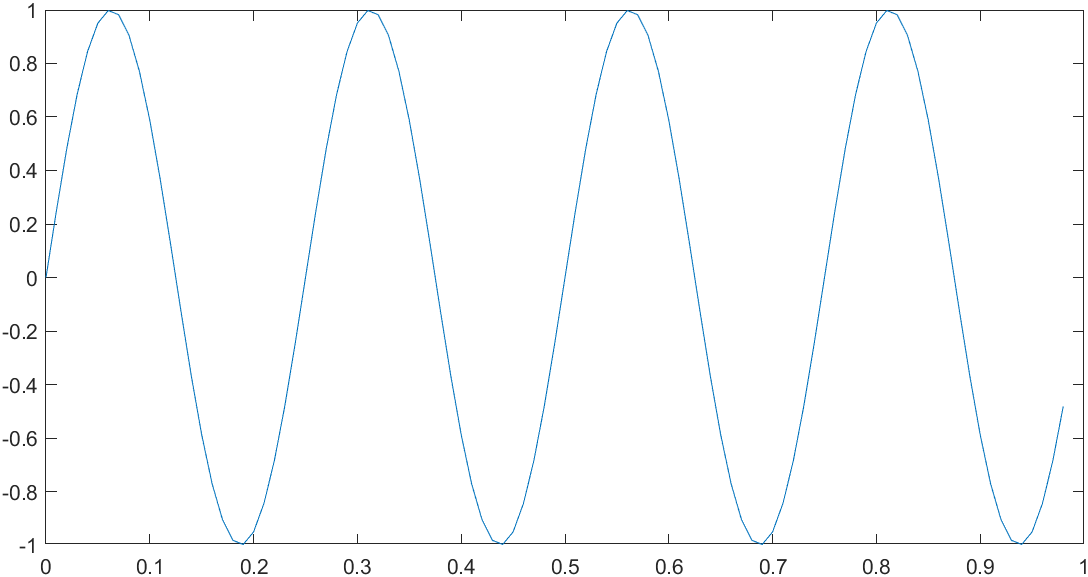``````>> y2 = cos(2*pi*4*t);
>> plot(t, y2)
``````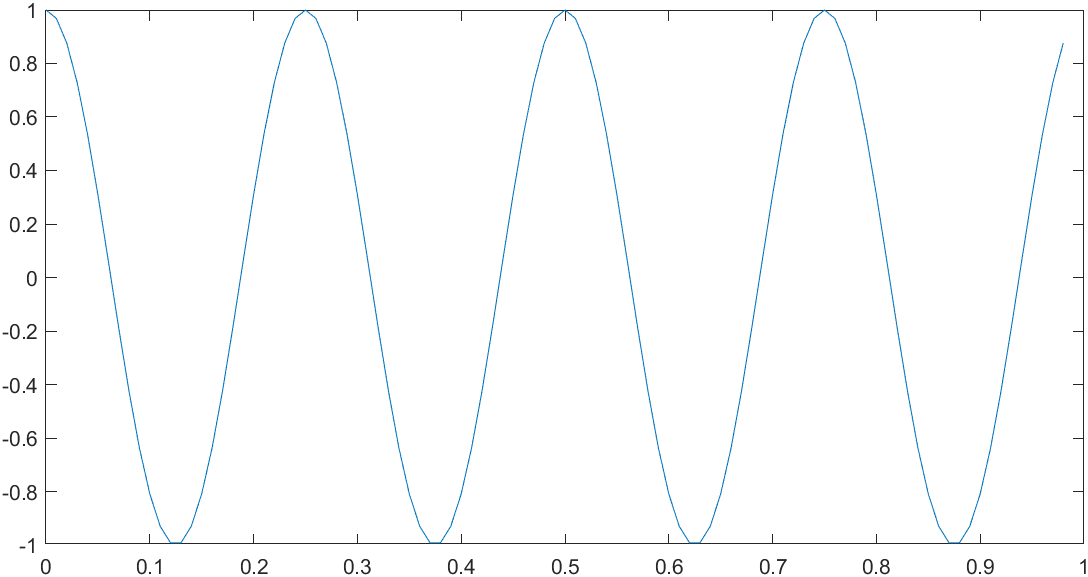‘r’ 为线条设定。每个设定可包含表示线条颜色、样式和标记的字符。标记是在绘制的每个数据点上显示的符号，例如，+、o 或 * ；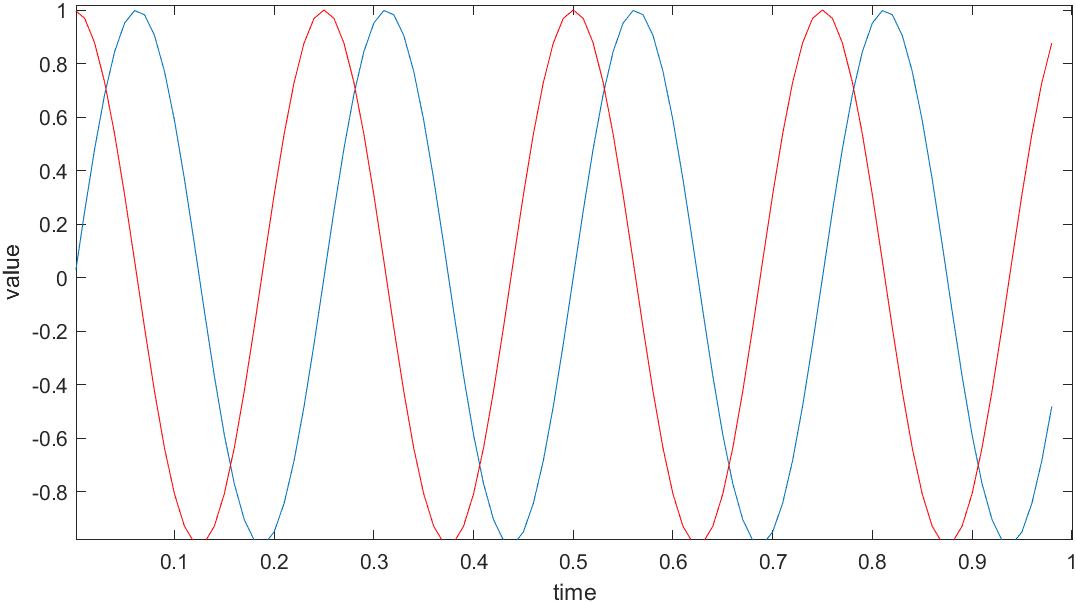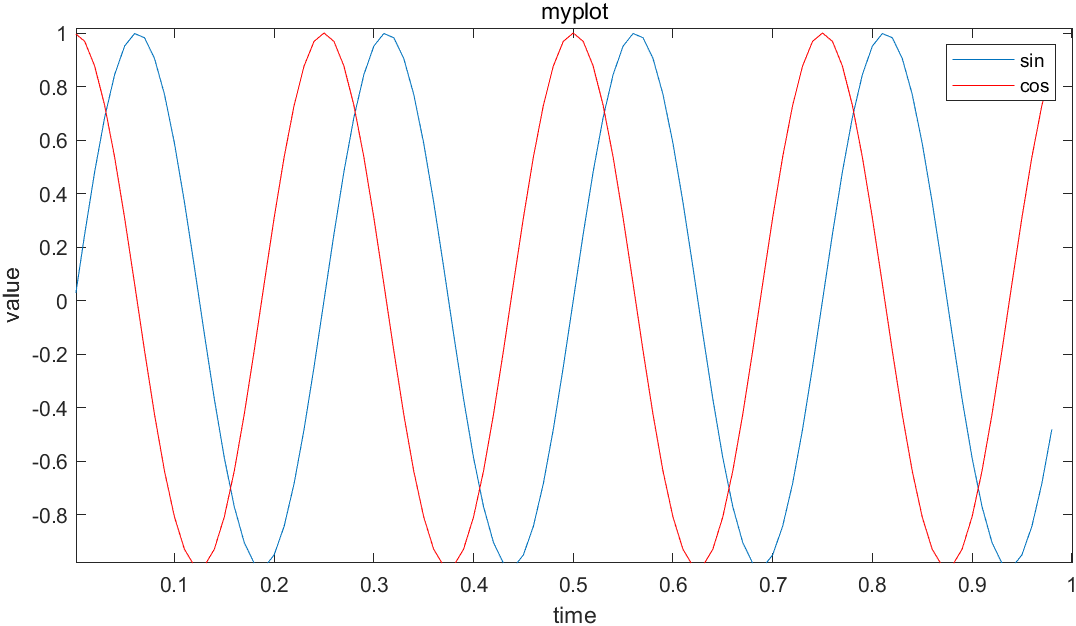• 使用`figure(1); plot(t, y1);`将显示第一张图，绘制了`y1-t`
• 使用`figure(2); plot(t, y2);`将显示第一张图，绘制了`y2-t`

`subplot`命令，我们使用`subplot(1,2,1)`，它将图像分为一个`1*2`的格子，也就是前两个参数，然后它使用第一个格子，也就是最后一个参数1的意思。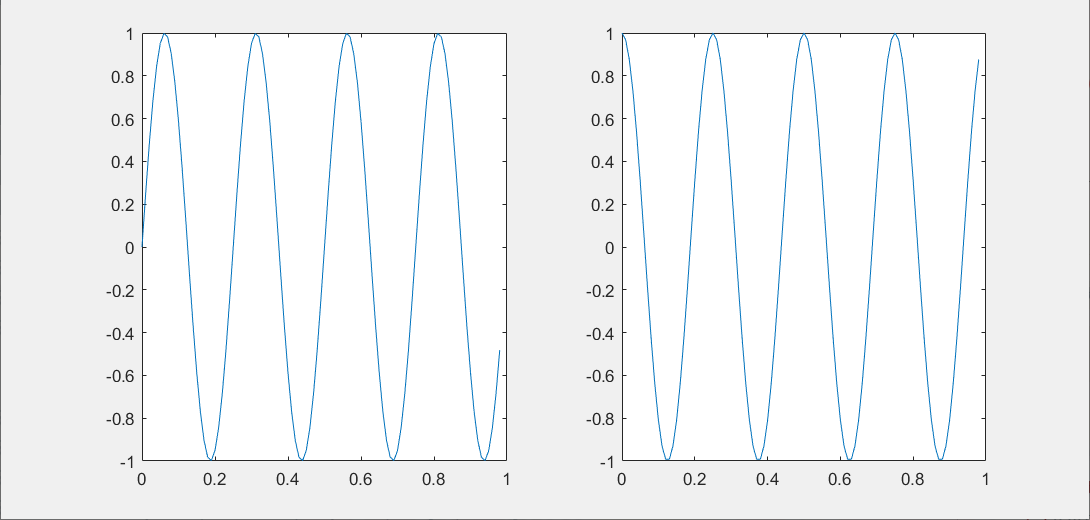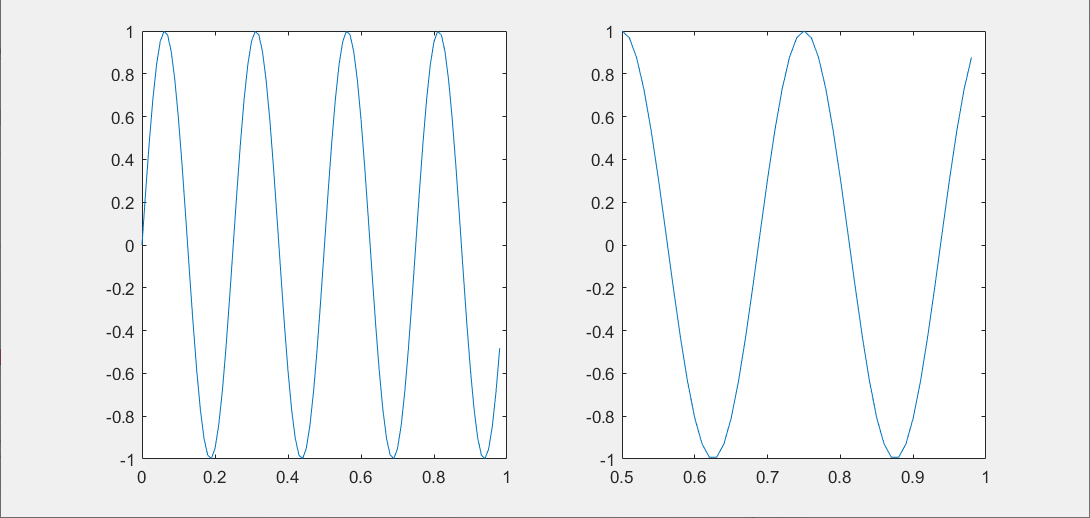`clf`命令可以清除当前的Figure

## imagesc

``````>> A = magic(5)
A =
17    24     1     8    15
23     5     7    14    16
4     6    13    20    22
10    12    19    21     3
11    18    25     2     9
``````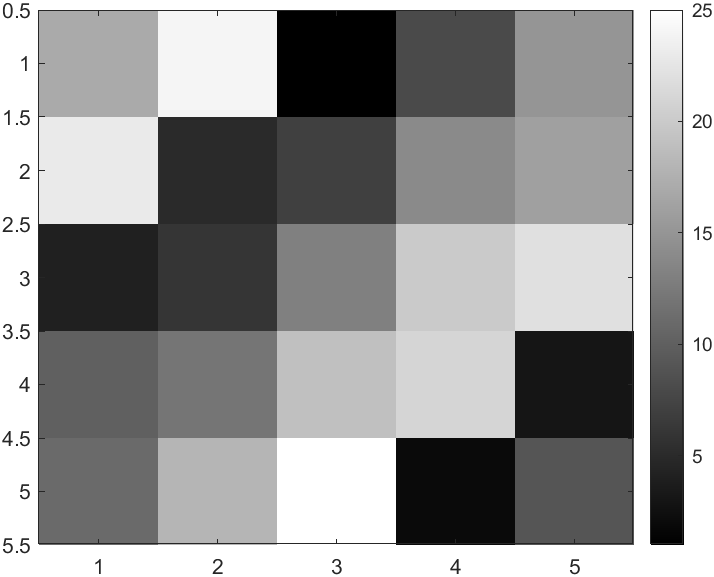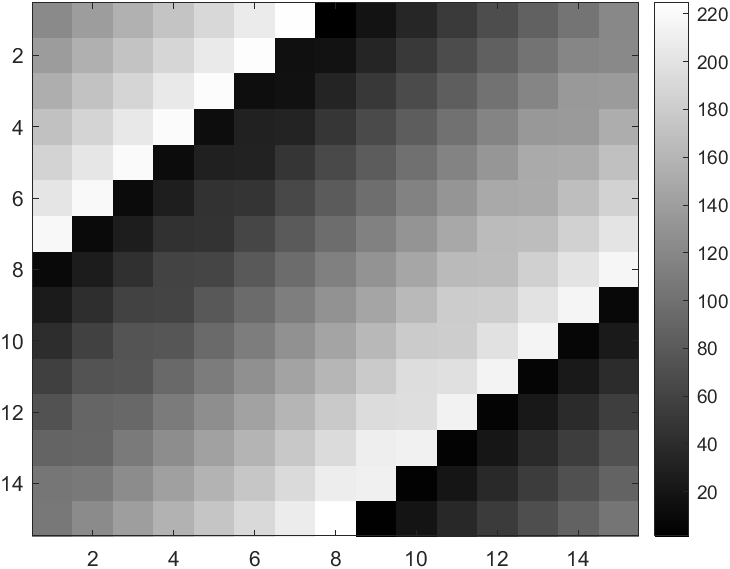## 三维绘图

``````>> [X,Y] = meshgrid(-2:.2:2);
>> Z = X .* exp(-X.^2 - Y.^2);
``````

meshgrid函数生成的X，Y是大小相等的矩阵

`surf(X, Y, Z)`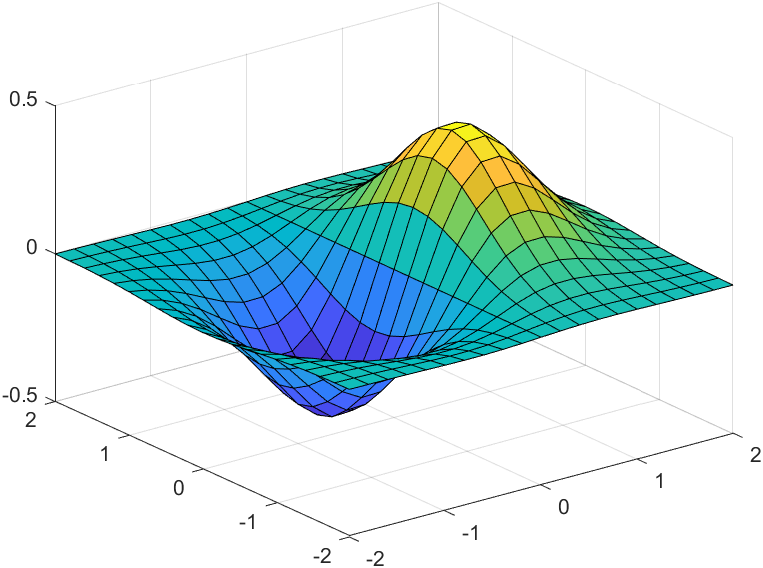surf 函数及其伴随函数 mesh 以三维形式显示曲面图。surf 使用颜色显示曲面图的连接线和面。mesh 生成仅以颜色标记连接定义点的线条的线框曲面图。